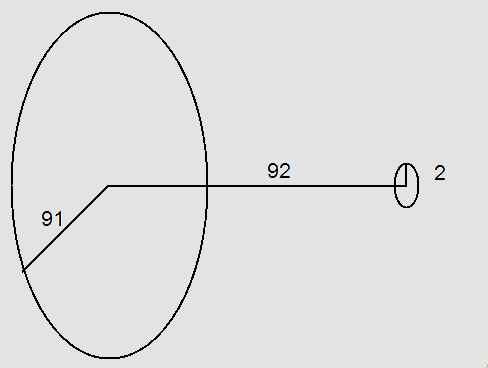# Mutual Inductance for a Pair of Coils

## Homework Statement

[URL]http://nplq1.phyast.pitt.edu/res/csm/csmphyslib/type66_inductance/PairOfCoils.jpg[/URL]
Coil A is a large 400 turn circular coil of radius 91.0 cm. Circular coil B has 160 turns, a radius of 2.0 cm and is located L = 92.0 cm from coil A along the same axis. The planes of the two coils are parallel. 1.) Find approximately the mutual inductance of this pair of coils. 2.) If the current in coil A varies with time according to I = 14t3 - 59t2 - 1, where I is in amps and t is in s, find the magnitude of the EMF induced in coil B at time t = 1.0 s.

N1 = 400 turns
N2 = 160 turns
R1 = .02 m
R2 = .91 m
L = .92 m
t = 1.0 s
I(t) = 14t3 - 59t2 - 1

## Homework Equations

$$\epsilon$$L = N1N2$$\Pi$$R22/2R1 * di(t)/dt

## The Attempt at a Solution

I attempted to solve for mutual inductance by: N1N2*pi*R22/2R1
And then multiplied by the derivative of I(t) at t=1.0s for the induced emf, but I'm not getting the correct answer. Any help or direction would be appreciated.

Last edited by a moderator:

Hi mjk71Welcome to PF !!

Was the mutual inductance you got correct?

Unfortunately, no.

Its really late here ... I'll answer your question in morning ..:zzz:

Hi mjk71The situation is somethinglike thislet there be a current i in bigger loop. find the field on axis of bigger loop at the distance where smaller loop is placed

find flux and then divide it by i
you'll get inductance M